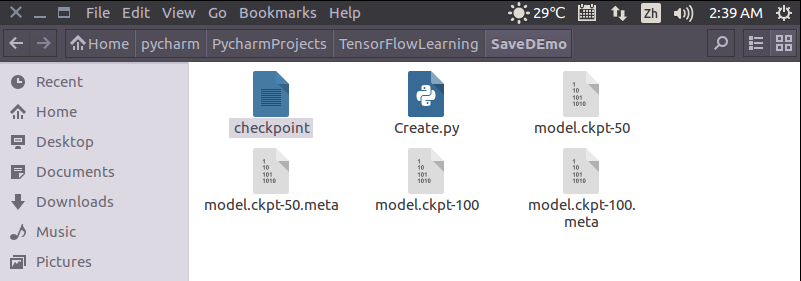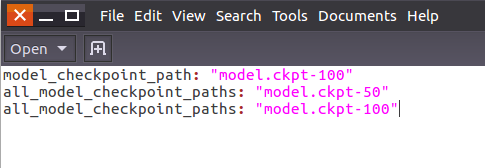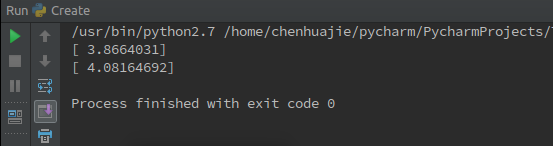# Tensorflow之Saver的用法详解

Saver的用法1.Saver的背景介绍我们经常在训练完一个模型之后希望保存训练的结果，这些结果

Saver的用法

1. Saver的背景介绍

Saver类提供了向checkpoints文件保存和从checkpoints文件中恢复变量的相关方法。Checkpoints文件是一个二进制文件，它把变量名映射到对应的tensor值 。

2. Saver的实例

``````
import tensorflow as tf
import numpy as np
x = tf.placeholder(tf.float32, shape=[None, 1])
y = 4 * x + 4
w = tf.Variable(tf.random_normal(, -1, 1))
b = tf.Variable(tf.zeros())
y_predict = w * x + b
loss = tf.reduce_mean(tf.square(y - y_predict))
train = optimizer.minimize(loss)
isTrain = False
train_steps = 100
checkpoint_steps = 50
checkpoint_dir = ''
saver = tf.train.Saver() # defaults to saving all variables - in this case w and b
x_data = np.reshape(np.random.rand(10).astype(np.float32), (10, 1))
with tf.Session() as sess:
sess.run(tf.initialize_all_variables())
if isTrain:
for i in xrange(train_steps):
sess.run(train, feed_dict={x: x_data})
if (i + 1) % checkpoint_steps == 0:
saver.save(sess, checkpoint_dir + 'model.ckpt', global_step=i+1)
else:
ckpt = tf.train.get_checkpoint_state(checkpoint_dir)
if ckpt and ckpt.model_checkpoint_path:
saver.restore(sess, ckpt.model_checkpoint_path)
else:
pass
print(sess.run(w))
print(sess.run(b)) ``````
1. isTrain：用来区分训练阶段和测试阶段，True表示训练，False表示测试
2. train_steps：表示训练的次数，例子中使用100
3. checkpoint_steps：表示训练多少次保存一下checkpoints，例子中使用50
4. checkpoint_dir：表示checkpoints文件的保存路径，例子中使用当前路径

2.1 训练阶段

1. sess：表示当前会话，当前会话记录了当前的变量值
2. checkpoint_dir + 'model.ckpt'：表示存储的文件名
3. global_step：表示当前是第几步2.1测试阶段

sess：表示当前会话，之前保存的结果将被加载入这个会话

ckpt.model_checkpoint_path：表示模型存储的位置，不需要提供模型的名字，它会去查看checkpoint文件，看看最新的是谁，叫做什么。